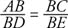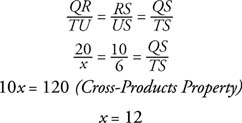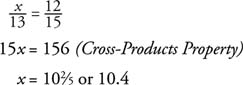## Proportional Parts of Triangles

Consider Figure 1 of Δ ABC with line l parallel to AC and intersecting the other two sides at D and E.Figure 1 Deriving the Side‐Splitter Theorem.

You can eventually prove that Δ ABC∼ Δ DBE using the AA Similarity Postulate. Because the ratios of corresponding sides of similar polygons are equal, you can show thatNow use Property 4, the Denominator Subtracion Property.But AB–DB = AD, and BC–BE = CE ( Segment Addition Postulate). With this replacement, you get the following proportion.This leads to the following theorem.

Theorem 57 (Side‐Splitter Theorem): If a line is parallel to one side of a triangle and intersects the other two sides, it divides those sides proportionally.

Example 1: Use Figure 2 to find x.Figure 2 Using the Side‐Splitter Theorem.

Because DE ‖ AC in Δ ABC by Theorem 57, you getExample 2: Use Figure 3 to find x.Figure 3 Using similar triangles.

Notice that TU , x, is not one of the segments on either side that TU intersects. This means that you cannot apply Theorem 57 to this situation. So what can you do? Recall that with TU ‖ QR , you can show that ΔQRS∼ Δ TUS. Because the ratios of corresponding sides of similar triangles are equal, you get the following proportion.Another theorem involving parts of a triangle is more complicated to prove but is presented here so you can use it to solve problems related to it.

Theorem 58 (Angle Bisector Theorem): If a ray bisects an angle of a triangle, then it divides the opposite side into segments that are proportional to the sides that formed the angle.

In Figure 4BD bisects ∠ ABC in Δ ABC. By Theorem 58,.Figure 4 Illustrating the Angle Bisector Theorem.

Example 3: Use Figure 5 to find x.Figure 5 Using the Angle Bisector Theorem.

Because BD bisects ∠ ABC in Δ ABC, you can apply Theorem 58.Back to Top
A18ACD436D5A3997E3DA2573E3FD792A